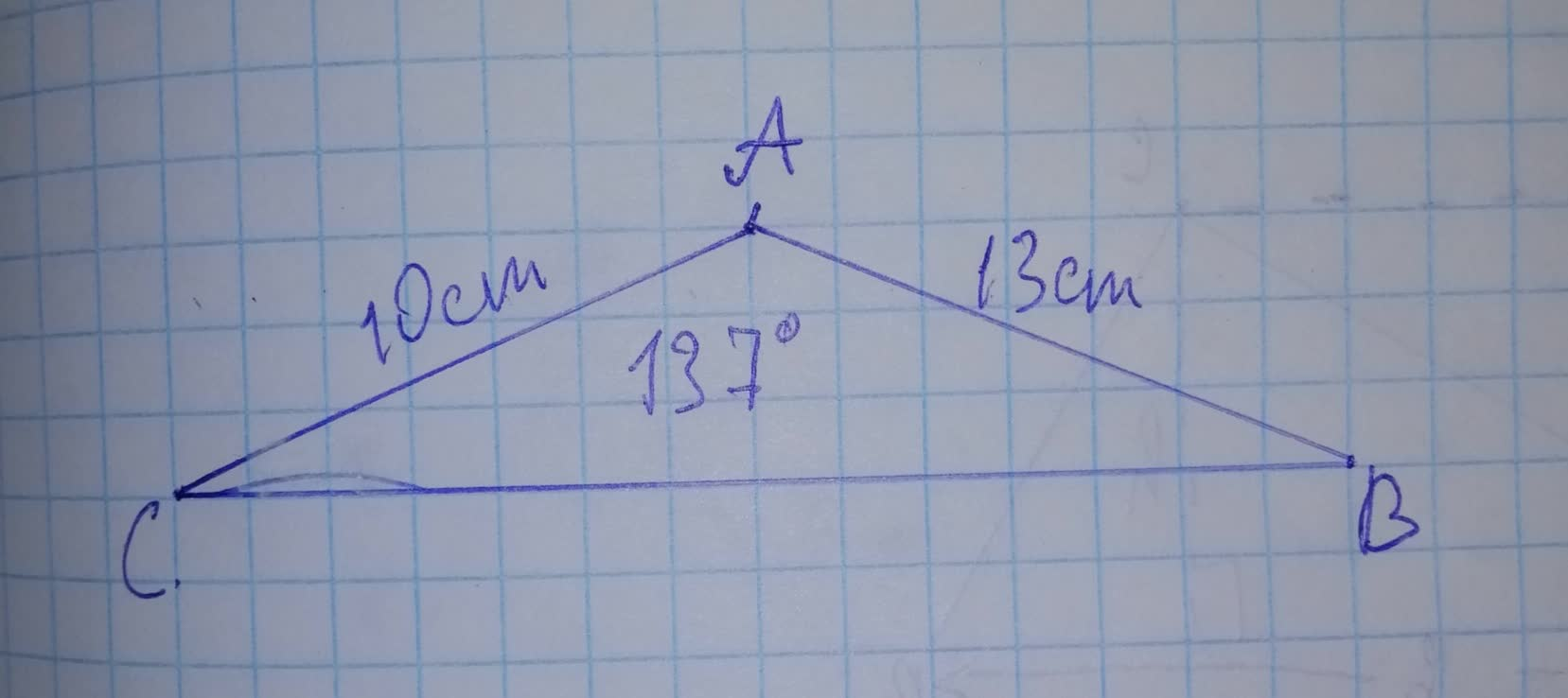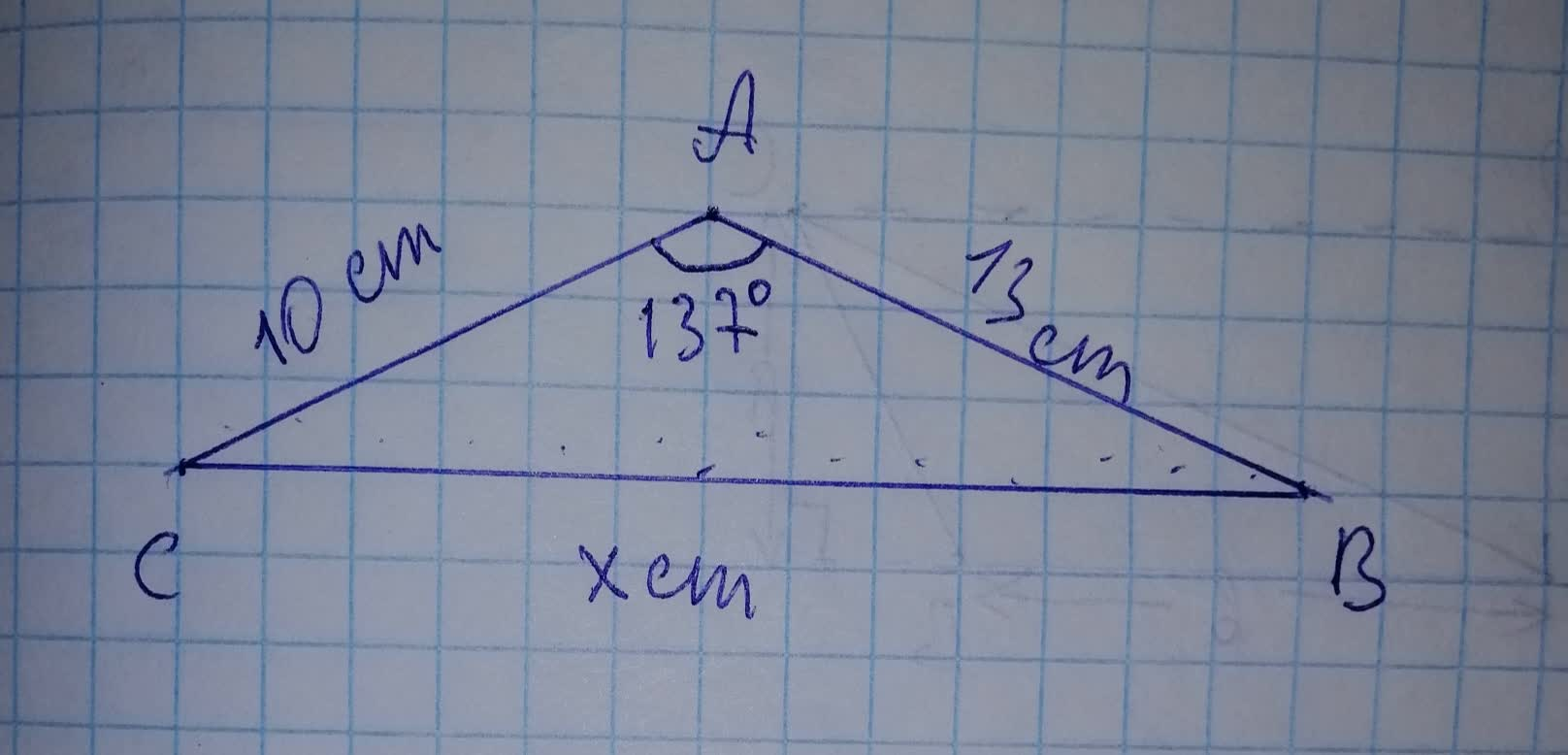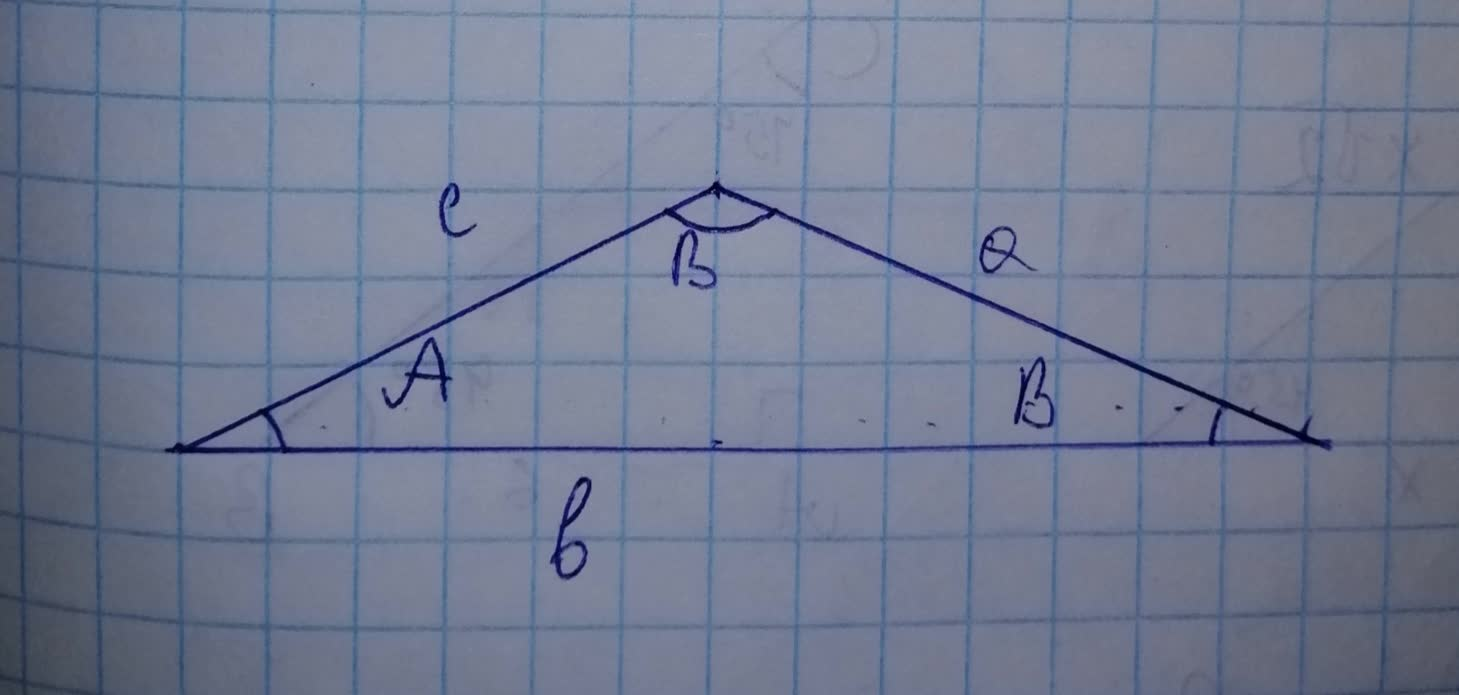# Find each measurement indicated. Round your answers to the nearest tenth. Fipamangking8 2021-11-18 Answered
Find BC• Questions are typically answered in as fast as 30 minutes

### Plainmath recommends

• Get a detailed answer even on the hardest topics.
• Ask an expert for a step-by-step guidance to learn to do it yourself.Kara Dillon

Step 1Since, we are given the length of the sides AC and AB withmeasurement of 10 cm and 13 cm of a non right angled triangles with $$\displaystyle{137}^{{\circ}}$$ between them
Our aim is to find the length of the side BC of a triangle i.e, to find the value of $$\displaystyle{x}=?$$
Step 2Using Cosine Rule in finding the unlown lenth of the side which states that:
For any non−right angled triangle with sides a, b and c:−
$$\displaystyle{b}^{{{2}}}={c}^{{{2}}}+{a}^{{{2}}}-{2}{c}{a}{\cos{{B}}}$$
$$\displaystyle\Rightarrow{x}^{{{2}}}={\left({10}\right)}^{{{2}}}+{\left({13}\right)}^{{{2}}}-{2}\times{10}\times{13}\times{\cos{{\left({137}^{{\circ}}\right)}}}$$
$$\displaystyle\Rightarrow{x}^{{{2}}}={100}+{169}-{\left({260}\times{0.334}\right)}$$
$$\displaystyle\Rightarrow{x}^{{{2}}}={269}-{86.88}$$
$$\displaystyle\Rightarrow{x}^{{{2}}}={182.12}$$
$$\displaystyle\Rightarrow{x}=\sqrt{{{182.12}}}$$
$$\displaystyle\Rightarrow{x}={13.49}{c}{m}$$
On rounding off to the nearest tenth we have $$\displaystyle{x}={13.5}{c}{m}$$
Therefore, the length of the side BC is 13.5 cm.